# Applied Survival Models

#### 2016-06-22

Survival modeling is a core component of any clinical data analysis toolset.

Here we will work through an example of fitting a survival model in Stan, using as an example data from TCGA on patients with Bladder Urothelial Carcinoma.

Fitting survival models in Stan is fairly straightforward. However, specifying a model in Stan requires a bit more thought than when using standard MLE tools such as survfit.

This vignette is part 1 of a multi-part series.

# Outline

Over the course of several vignettes, we will review various models and approaches to analysis.

In this vignette, we cover the following:

1. Data review & inspection
2. Review parametric Weibull survival model
3. Test parametric survival model against simulated data
4. Fit NULL parametric survival model with TCGA data
5. Check convergence & review posterior predictive checks on model

Part II of this series will consider a non-parametric piecewise exponential model.

# Data

We will use data from The Cancer Genome Atlas (TCGA) for patients diagnosed with Bladder Urothelial Carcinoma. This cohort has an acronym BLCA.

TCGA is a repository giving access to clinical and molecular data for a variety of cancer types, primarily focusing on genomic datasets and outcomes following standard therapeutic interventions. The BLCA cohort is described in this this paper.

The complete clinical & molecular data are available from TCGA data portals, but in this case we will use a curated version of these data available from http://www.cbioportal.org/.

To load the data into R, we could query these data via the web-url service:

url <- 'http://www.cbioportal.org/webservice.do?cmd=getClinicalData&case_set_id=blca_tcga_all'
req <- httr::GET(url)
clinical_data <-
httr::content(req,
type = 'text/tab-separated-values',
col_names = T,
col_types = NULL
)
str(clinical_data)

However, MSKCC has provided the CGDS-R package, which provides an easier interface to the same data.

Run the following lines of code to load the clinical data for this cohort:

mycgds = cgdsr::CGDS("http://www.cbioportal.org/public-portal/")
selected_case_list = 'blca_tcga_all'
clinical_data = cgdsr::getClinicalData(mycgds, selected_case_list)

Describe the structure of data available:

str(clinical_data,  no.list = T, vec.len = 2)
## 'data.frame':    413 obs. of  75 variables:
##  $AGE : int 77 73 67 84 78 ... ##$ AJCC_METASTASIS_PATHOLOGIC_PM             : chr  "MX" "M0" ...
##  $AJCC_NODES_PATHOLOGIC_PN : chr "N0" "N0" ... ##$ AJCC_PATHOLOGIC_TUMOR_STAGE               : chr  "Stage II" "Stage III" ...
##  $AJCC_STAGING_EDITION : chr "7th" "7th" ... ##$ AJCC_TUMOR_PATHOLOGIC_PT                  : chr  "T2a" "T3" ...
##  $ANGIOLYMPHATIC_INVASION : chr "NO" "NO" ... ##$ CLIN_T_STAGE                              : chr  "" "" ...
##  $DAYS_TO_BIRTH : int -28204 -27000 -24625 -30686 -28757 ... ##$ DAYS_TO_COLLECTION                        : int  99 149 84 447 1157 ...
##  $DAYS_TO_DEATH : int NA NA NA 328 NA ... ##$ DAYS_TO_INITIAL_PATHOLOGIC_DIAGNOSIS      : int  0 0 0 0 0 ...
##  $DAYS_TO_LAST_FOLLOWUP : int 162 28 0 NA 1248 ... ##$ DFS_MONTHS                                : num  19.05 0.92 ...
##  $DFS_STATUS : chr "DiseaseFree" "DiseaseFree" ... ##$ ECOG_SCORE                                : chr  "" "1" ...
##  $ETHNICITY : chr "NOT HISPANIC OR LATINO" "NOT HISPANIC OR LATINO" ... ##$ FAMILY_HISTORY_CANCER_RELATIONSHIP        : chr  "" "" ...
##  $FAMILY_HISTORY_CANCER_TYPE : chr "" "" ... ##$ FORM_COMPLETION_DATE                      : chr  "2012-8-27" "2013-12-16" ...
##  $GENDER : chr "MALE" "MALE" ... ##$ HEIGHT                                    : num  180 173 170 188 NA ...
##  $HISTOLOGICAL_SUBTYPE : chr "Non-Papillary" "Papillary" ... ##$ HISTORY_NEOADJUVANT_TRTYN                 : chr  "No" "No" ...
##  $HISTORY_OTHER_MALIGNANCY : chr "Yes" "Yes" ... ##$ ICD_10                                    : chr  "C67.9" "C67.0" ...
##  $ICD_O_3_HISTOLOGY : chr "8120/3" "8120/3" ... ##$ ICD_O_3_SITE                              : chr  "C67.9" "C67.0" ...
##  $INFORMED_CONSENT_VERIFIED : chr "YES" "YES" ... ##$ INITIAL_PATHOLOGIC_DX_YEAR                : int  2012 2013 2012 2011 2009 ...
##  $INITIAL_WEIGHT : int 500 1470 320 910 480 ... ##$ IS_FFPE                                   : chr  "NO" "NO" ...
##  $KARNOFSKY_PERFORMANCE_SCORE : chr "90" "80" ... ##$ LYMPH_NODES_EXAMINED                      : chr  "YES" "YES" ...
##  $LYMPH_NODES_EXAMINED_HE_COUNT : int 0 0 NA 0 0 ... ##$ LYMPH_NODE_EXAMINED_COUNT                 : int  19 14 NA 40 19 ...
##  $METHOD_OF_INITIAL_SAMPLE_PROCUREMENT : chr "Transurethral resection (TURBT)" "Transurethral resection (TURBT)" ... ##$ METHOD_OF_INITIAL_SAMPLE_PROCUREMENT_OTHER: chr  "" "" ...
##  $NEW_TUMOR_EVENT_AFTER_INITIAL_TREATMENT : chr "NO" "NO" ... ##$ NONINVASIVE_BLADDER_CA_TX_TYPE            : chr  "" "Transurethral resection alone" ...
##  $NONINVASIVE_BLADDER_HISTORY : chr "YES" "YES" ... ##$ OCCUPATION_CURRENT                        : chr  "" "Retired" ...
##  $OCCUPATION_PRIMARY : chr "" "" ... ##$ OCCUPATION_PRIMARY_CHEMICAL_EXPOSURE      : chr  "Secondhand" "" ...
##  $OCCUPATION_PRIMARY_INDUSTRY : chr "" "" ... ##$ OCCUPATION_PRIMARY_YEARS_WORKED           : int  NA NA NA NA NA ...
##  $OCT_EMBEDDED : chr "true" "true" ... ##$ OS_MONTHS                                 : num  19.05 0.92 ...
##  $OS_STATUS : chr "LIVING" "LIVING" ... ##$ OTHER_PATIENT_ID                          : chr  "10422FE9-8D70-40A9-9875-3FE9FAB68823" "6F30B069-C8F5-424C-9FB5-F7DBA508CB8B" ...
##  $OTHER_SAMPLE_ID : chr "FE3F7C73-8E04-48BA-AF35-E369F5C62E98" "784E85BB-9C4C-44B4-8BFC-3B2DBC1406C8" ... ##$ PATHOLOGY_REPORT_FILE_NAME                : chr  "TCGA-GV-A40G.28754343-DA2B-4608-A66C-B4E41B52F6FF.pdf" "TCGA-E7-A7DU.BEB0E95C-EDF2-40A5-BB95-588D07369CEE.pdf" ...
##  $PATHOLOGY_REPORT_UUID : chr "28754343-DA2B-4608-A66C-B4E41B52F6FF" "BEB0E95C-EDF2-40A5-BB95-588D07369CEE" ... ##$ PHARMACEUTICAL_TX_ADJUVANT                : chr  "NO" "[Unknown]" ...
##  $PROSPECTIVE_COLLECTION : chr "YES" "YES" ... ##$ RACE                                      : chr  "WHITE" "WHITE" ...
##  $RADIATION_TREATMENT_ADJUVANT : chr "NO" "NO" ... ##$ RETROSPECTIVE_COLLECTION                  : chr  "NO" "NO" ...
##  $SAMPLE_TYPE : chr "Primary Tumor" "Primary Tumor" ... ##$ SAMPLE_TYPE_ID                            : int  1 1 1 1 1 ...
##  $SMOKING_PACK_YEARS : num 30 NA 30 40 20 ... ##$ SMOKING_YEAR_STARTED                      : int  18 NA 37 NA NA ...
##  $SMOKING_YEAR_STOPPED : int 1997 1980 2012 1992 1950 ... ##$ TIME_TO_COMPLETE_RESPONSE                 : int  NA NA NA NA NA ...
##  $TISSUE_SOURCE_SITE : chr "GV" "E7" ... ##$ TOBACCO_SMOKING_HISTORY_INDICATOR         : chr  "4" "3" ...
##  $TREATMENT_OUTCOME_FIRST_COURSE : chr "Complete Remission/Response" "Complete Remission/Response" ... ##$ TUMOR_STATUS                              : chr  "TUMOR FREE" "TUMOR FREE" ...
##  $TX_90DAYS_PRIOR_TO_RESECTION : chr "" "" ... ##$ TX_COMPLETE_RESPONSE                      : chr  "" "" ...
##  $TX_INDUCTION_COURSES_INDICATOR : chr "" "" ... ##$ TX_MAINTENANCE_COURSES_INDICATOR          : chr  "" "" ...
##  $VIAL_NUMBER : chr "A" "A" ... ##$ VITAL_STATUS                              : chr  "Alive" "Alive" ...
##  $WEIGHT : num 82 110 52 80 NA ... ## Data cleaning Let’s do some minimal data manipulation on this DataFrame to make it easier to work with. I personally happen to like lower-case variable names and to work with NA values instead of empty strings. We will save the converted data in a data.frame called clin_data. ## names to lower case names(clinical_data) <- tolower(names(clinical_data)) ## convert empty strings -> NA values convert_blank_to_na <- function(x) { if (!purrr::is_character(x)) { warning('input vector is not character - returning original input') return(x) } else { ifelse(x == '', NA, x) } } clin_data <- clinical_data %>% dplyr::mutate_each(funs = funs(convert_blank_to_na), everything()) ## inspect resulting data frame str(clin_data, vec.len = 2, list.len = 10) ## 'data.frame': 413 obs. of 75 variables: ##$ age                                       : int  77 73 67 84 78 ...
##  $ajcc_metastasis_pathologic_pm : chr "MX" "M0" ... ##$ ajcc_nodes_pathologic_pn                  : chr  "N0" "N0" ...
##  $ajcc_pathologic_tumor_stage : chr "Stage II" "Stage III" ... ##$ ajcc_staging_edition                      : chr  "7th" "7th" ...
##  $ajcc_tumor_pathologic_pt : chr "T2a" "T3" ... ##$ angiolymphatic_invasion                   : chr  "NO" "NO" ...
##  $clin_t_stage : chr NA NA ... ##$ days_to_birth                             : int  -28204 -27000 -24625 -30686 -28757 ...
##  $days_to_collection : int 99 149 84 447 1157 ... ## [list output truncated] ## Data exploration In survival analysis, the outcome or dependent variable is the time to event where some event times are not observed (IE they are censored). Here we consider the more common scenario of right-censoring. This is the case where the terminating event is not observed. Observations are instead censored at time t . Our first analysis will treat overall survival as the event of interest, as opposed to progression-free survival. In this cohort, the overall survival is described by two variables: os_status & os_months. We will start by inspecting these data. ### Negative/missing event times We have one observation with missing data for os_status: clinical_data %>% dplyr::filter(is.na(os_status) | os_status == '') %>% dplyr::select(os_status, os_months) %>% str() ## 'data.frame': 1 obs. of 2 variables: ##$ os_status: chr ""
##  $os_months: num NA We have a 3 observations with unknown and/or negative survival times (os_months < 0): clinical_data %>% dplyr::filter(!is.na(os_status) & os_status != '') %>% dplyr::filter(os_months < 0 | is.na(os_months)) %>% dplyr::select(os_status, os_months) %>% head() ## os_status os_months ## 1 DECEASED NA ## 2 DECEASED NA ## 3 LIVING -2.1 For now, we remove these observations from our analysis dataset (clin_data). clin_data <- clin_data %>% dplyr::filter(!is.na(os_status) & os_status != '') %>% dplyr::filter(os_months >= 0 & !is.na(os_months)) ## confirm 4 fewer observations than original assert_that(nrow(clin_data) == nrow(clinical_data) - 4) ##  TRUE ### Distribution of event times Among the remaining observations, the median time to event is 17.61 months. Event times are distributed as follows among observed (DECEASED) & censored (LIVING) observations: ggplot(clin_data, aes(x = os_months, group = os_status, colour = os_status, fill = os_status )) + geom_density(alpha = 0.5)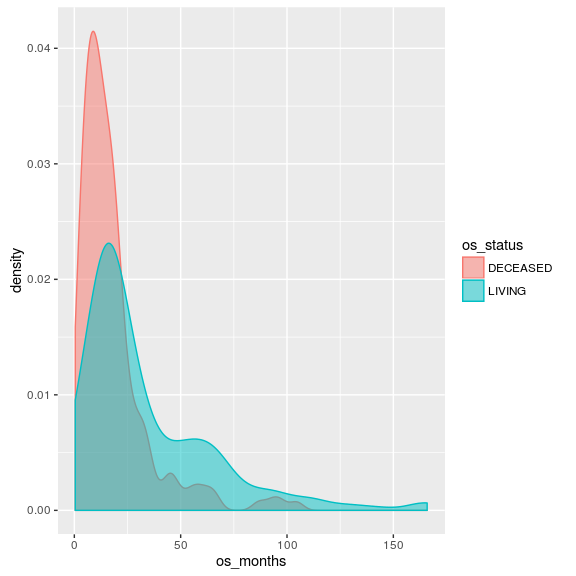The KM curve for these observations additionally looks like this: ( constructed using survfit ) mle.surv <- survfit( Surv(os_months,os_deceased) ~ 1, data = clin_data %>% dplyr::mutate(os_deceased = os_status == 'DECEASED') ) autoplot(mle.surv, conf.int = F) + ggtitle('KM survival curve for BLCA cohort')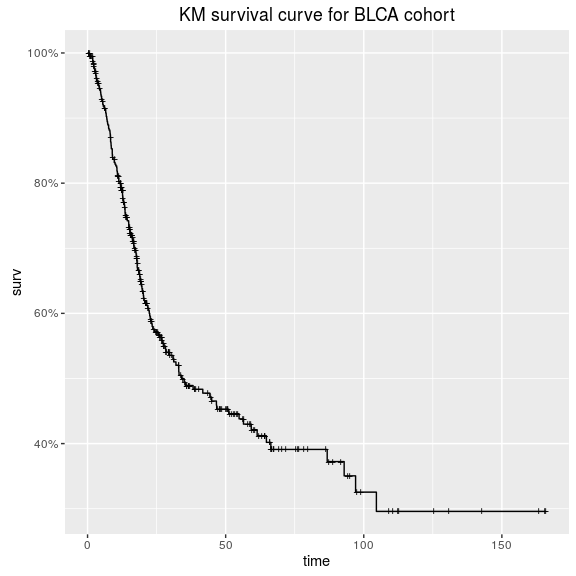# First analysis: parametric survival model For our first analysis we will work with a parametric Weibull survival model. We will start with model code adapted from wei_bg.stan within the github repo accompanying Peltola et al, 2014’s nice paper describing a bayesian approach to biomarker evaluation. This model assumes that the time to event x follows a Weibull distribution. Stan parameterizes this probability density function as : $f(x|\alpha,\sigma) = \frac{\alpha}{\sigma}\left(\frac{x}{\sigma}\right)^{\alpha-1}e^{-(x/\sigma)^{\alpha}}$ In the context of this analysis, we will define two parameters: • alpha (shape) defined as above • mu (scale) where $$\sigma = e^\frac{-\mu}{\alpha}$$. If we had covariates and wanted to estimate a proportional hazards model, we would replace mu with a linear combination of covariates. However, in this case we are interested in recovering features of our NULL model and so we treat mu as a constant intercept. ## Stan code for the model The stan code for this model is included in this biostan package as weibull_survival_null_model.stan. It can be accessed by calling system.file(), as: if (!require(biostan)) devtools::install_github('jburos/biostan') library(biostan) stan_file <- system.file('stan', 'weibull_survival_null_model.stan', package = 'biostan') Here are the contents of this file: biostan::print_stan_file(stan_file) ## /* Variable naming: ## obs = observed ## cen = (right) censored ## N = number of samples ## tau = scale parameter ## */ ## data { ## int<lower=0> Nobs; ## int<lower=0> Ncen; ## vector[Nobs] yobs; ## vector[Ncen] ycen; ## } ## ## transformed data { ## real<lower=0> tau_mu; ## real<lower=0> tau_al; ## ## tau_mu <- 10.0; ## tau_al <- 10.0; ## } ## ## parameters { ## real alpha_raw; ## real mu; ## } ## ## transformed parameters { ## real alpha; ## alpha <- exp(tau_al * alpha_raw); ## } ## ## model { ## yobs ~ weibull(alpha, exp(-(mu)/alpha)); ## increment_log_prob(weibull_ccdf_log(ycen, alpha, exp(-(mu)/alpha))); ## ## alpha_raw ~ normal(0.0, 1.0); ## mu ~ normal(0.0, tau_mu); ## } ##  ### The model in detail Before using this model for analysis, we want to first review the model code in detail & test it against some simulated data. This will ensure that (a) we understand the model well, and (b) the model can recover estimates from simulated data. ( As you will see, several parts of the simulate-data process can also be re-used for posterior predictive checking. So we will save components of the process to be reused in later steps. ) If you’re at an R console, you can open the Stan file in an editor as follows: if (interactive()) file.edit(stan_file) #### Review data block Let’s review the data block first. This will tell us the structure and format of data input to the model. print_stan_file(stan_file, section = 'data') ## data { ## int<lower=0> Nobs; ## int<lower=0> Ncen; ## vector[Nobs] yobs; ## vector[Ncen] ycen; ## } The censored & observed data points are provided as separate input vectors. observed data points • Nobs: number of observed data points • yobs: times to observed events censored data points • Ncen: number of censored data points • ycen: times to censored events Recall that this is a NULL model (with no covariate values), so the number & values of observed covariates are not needed. #### Review model block The stan code contains an implicit constant term, in the linear predictor mu. print_stan_file(stan_file, section = 'model') ## model { ## yobs ~ weibull(alpha, exp(-(mu)/alpha)); ## increment_log_prob(weibull_ccdf_log(ycen, alpha, exp(-(mu)/alpha))); ## ## alpha_raw ~ normal(0.0, 1.0); ## mu ~ normal(0.0, tau_mu); ## } Observe how the ccdf (complementary cumulative distribution function) is used to compute the log probability of the censored observations. What does the ccdf represent in this scenario? How does the model address the censoring process? #### Review parameters block Our stan code also contains a reparameterization of the alpha term, in the transformed parameters block. Observe: print_stan_file(stan_file, section = 'transformed parameters') ## transformed parameters { ## real alpha; ## alpha <- exp(tau_al * alpha_raw); ## } (recall that tau_al is a constant scaling term set to 10, and alpha_raw is a parameter with a normal(0, 1) prior distribution). This reparameterization achieves two things : 1. The use of tau_al * alpha_raw is an example of a non-centered parameterization. • It would have been mathematically equivalent to define a (non-transformed) parameter alpha with a prior normal(0, 10). • However, this parameterization yields a parameter (alpha_raw) which is on a similar scale as other parameters in our model. The exp() transformation makes the difference between these two scales even more dramatic. • In general, having all parameters on a similar scale makes the sampling more efficient. 2. The exp() transformation of this parameter additionally allows us to put a prior on log alpha. • we want to put a prior on log alpha since alpha enters into our likelihood in the exponent. This seems like a lot of gymnastics to be doing. However, it has practical implications for our modeling efficiency. Observe that, for a single value of alpha_raw (e.g. 0.2), the transformation yields: alpha_raw <- 0.2 tau_al <- 10 log_alpha <- alpha_raw * tau_al alpha <- exp(log_alpha) print(alpha) ##  7.389056 which may seem silly. However Consider the resulting distribution of alpha over a range of values for alpha_raw sampled from our normal(0, 1) prior: alpha_raw <- rnorm(1000, 0, 1) tau_al <- 10 log_alpha <- alpha_raw * tau_al alpha <- exp(log_alpha) ggplot(data.frame(alpha = alpha, alpha_raw = alpha_raw), aes(x = alpha)) + geom_density() + scale_x_log10(labels = scientific)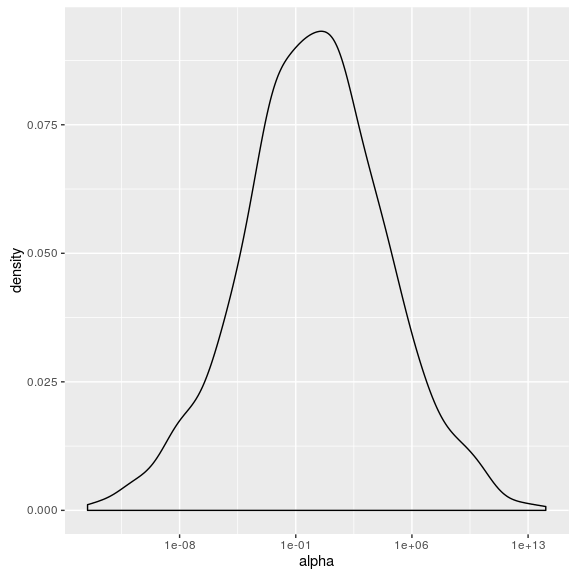Notice how alpha ranges from 1e-10 to 1e+10 on a log scale. We have to truncate this dramatically to even consider plotting it on its original scale. Sampling this parameter space may require different step sizes & different tuning parameter values throughout this distribution. The alpha_raw scale, by comparison, is a lot friendlier. ggplot(data.frame(alpha = alpha, alpha_raw = alpha_raw), aes(x = alpha, y = alpha_raw)) + geom_density2d() + scale_x_log10(labels = scientific)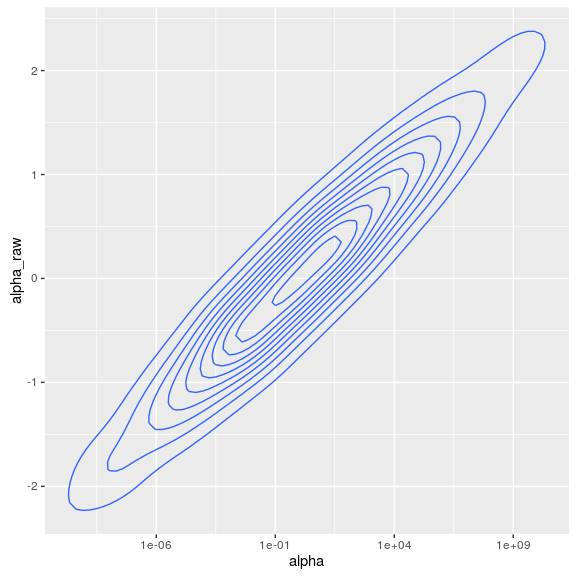This distribution is centered at 0 and has more consistent behavior throughout its range of values. What’s important to note here is that while the non-centered parameterization is mathematically equivalent to the standard parameterization, it is (in some ways) a different model. Consider that the reparameterization will impact the posterior estimates of parameter values. Packages like rstanarm which provide easy wrappers to a variety of standard models implemented in Stan use a non-centered parameterization by default. More information on non-centered parameterization: ## Testing the model on simulated data Now that we have reviewed the model code, we are ready to simulate data according to this model. We can simulate data using R or in Stan. We will start by simulating data in R. ### Simulate data in R Like our stan model code, we originally based this function on that used by the example.R file from the stan-survival-shrinkage github repo. However, after further inspection (see the related weibull-survival-model vignette) we modified the simulate-data function slightly. Here we will work with the modified function. Our weibull_sim_data function takes two parameters (alpha and mu) as inputs and a desired sample size (n). It returns a data frame of simulated event times. weibull_sim_data <- function(alpha, mu, n) { data <- data.frame(surv_months = rweibull(n = n, alpha, exp(-(mu)/alpha)), censor_months = rexp(n = n, rate = 1/100), stringsAsFactors = F ) %>% dplyr::mutate(os_status = ifelse(surv_months < censor_months, 'DECEASED', 'LIVING' ), os_months = ifelse(surv_months < censor_months, surv_months, censor_months ) ) return(data) } A few comments about this function: 1. Notice how the censoring process is rexp(). We chose this somewhat arbitrarily. • In general, our Stan model is ignorant of the censoring process except to assume that censoring is noninformative. 2. We have also deliberately written this function to mimic the structure of our clinical data. This will make it easier to reuse this & other functions later. #### Simulate data for arbitrary input values We can use this function to simulate a dataset for hypothetical parameter values of alpha & mu. test_alpha <- 0.8 test_mu <- -3 ## sample size from TCGA blca data test_n <- nrow(clin_data) ## test these inputs for arbitrary values of alpha & mu simulated_data <- weibull_sim_data(alpha = test_alpha, mu = test_mu, n = test_n ) head(simulated_data) ## surv_months censor_months os_status os_months ## 1 0.5995253 15.65978 DECEASED 0.5995253 ## 2 24.4544603 62.24349 DECEASED 24.4544603 ## 3 5.9572821 87.10862 DECEASED 5.9572821 ## 4 29.3960125 32.69540 DECEASED 29.3960125 ## 5 19.1953454 94.13512 DECEASED 19.1953454 ## 6 207.2250962 343.31931 DECEASED 207.2250962 The simulated survival curve looks like: ## plot KM curve from simulated data simulated_data <- simulated_data %>% dplyr::mutate(os_deceased = os_status == 'DECEASED') autoplot(survival::survfit(Surv(os_months, os_deceased) ~ 1, data = simulated_data ), conf.int = F) + ggtitle('Simulated KM curve')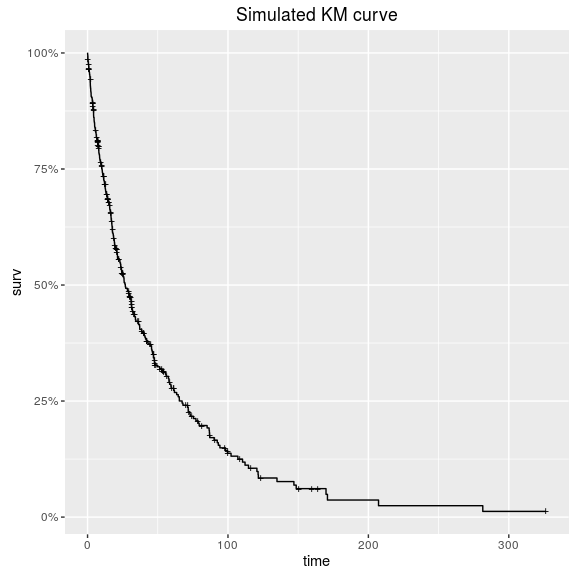### fit to simulated data in stan Now that we have simulated data, we are ready to fit the model in Stan. If we have written both our stan code & simulated data process correctly, our posterior intervals for alpha and mu should contain the values used to simulate our dataset (0.8 and -3). #### preparing data for stan Stan takes data input as a list. The contents of the list should match those of the data block in the stan code. E.g. looking at the data block - print_stan_file(stan_file, section = 'data') ## data { ## int<lower=0> Nobs; ## int<lower=0> Ncen; ## vector[Nobs] yobs; ## vector[Ncen] ycen; ## } our input list to Stan should contain dimensions & values for observed & censored data, separately. observed_data <- simulated_data %>% dplyr::filter(os_status == 'DECEASED') censored_data <- simulated_data %>% dplyr::filter(os_status != 'DECEASED') stan_data <- list( Nobs = nrow(observed_data), Ncen = nrow(censored_data), yobs = observed_data$os_months,
ycen = censored_data$os_months ) rm(censored_data) rm(observed_data) str(stan_data) ## List of 4 ##$ Nobs: int 292
##  $Ncen: int 117 ##$ yobs: num [1:292] 0.6 24.45 5.96 29.4 19.2 ...
##  $ycen: num [1:117] 33.17 9.95 17.57 6.31 150.28 ... (wrap this prep-data process in a function gen_stan_data for later) gen_stan_data <- function(data) { observed_data <- data %>% dplyr::filter(os_status == 'DECEASED') censored_data <- data %>% dplyr::filter(os_status != 'DECEASED') stan_data <- list( Nobs = nrow(observed_data), Ncen = nrow(censored_data), yobs = observed_data$os_months,
ycen = censored_data$os_months ) } #### test simulated values with stan Let’s call stan: recover_simulated <- rstan::stan(stan_file, data = gen_stan_data(simulated_data), chains = 4, iter = 1000, seed = 1328025050 ) print(recover_simulated) ## Inference for Stan model: weibull_survival_null_model. ## 4 chains, each with iter=1000; warmup=500; thin=1; ## post-warmup draws per chain=500, total post-warmup draws=2000. ## ## mean se_mean sd 2.5% ## alpha_raw 6.000000e-02 9.000000e-02 1.300000e-01 -3.000000e-02 ## mu -2.630000e+00 7.100000e-01 1.010000e+00 -3.500000e+00 ## alpha 5.210000e+00 5.350000e+00 7.570000e+00 7.600000e-01 ## lp__ -1.220654e+45 1.494616e+45 2.114767e+45 -4.892679e+45 ## 25% 50% 75% 97.5% n_eff Rhat ## alpha_raw -2.000000e-02 -0.02 0.07 0.29 2 33.82 ## mu -3.270000e+00 -3.14 -2.17 -0.89 2 7.38 ## alpha 8.200000e-01 0.86 5.31 18.32 2 228.85 ## lp__ -1.217518e+45 -1400.52 -1399.71 -1399.34 2 1240.90 ## ## Samples were drawn using NUTS(diag_e) at Wed Jun 22 22:20:40 2016. ## For each parameter, n_eff is a crude measure of effective sample size, ## and Rhat is the potential scale reduction factor on split chains (at ## convergence, Rhat=1). What’s wrong with this picture? (A: poor convergence) (A: in some chains, we see a lot of numerical problems.) #### Setting initial values This step is usually optional, but may be necessary for some models. In this case, it may be useful to set initial values. Recall the projected range of our transformed parameter alpha? By default, Stan chooses a random initial value for each parameter on the unconstrained scale between -2 and 2. This random initialization is on the unconstrained support for each parameter. This guarantees that initial values are consistent with the constrained range. When we pass the initial values in, however, these are on the constrained scale. See the Stan manual for more details about transformations applied to constrained variables. ##### gen_inits function Let’s review the parameters block for this model again. print_stan_file(stan_file, section = 'parameters') ## parameters { ## real alpha_raw; ## real mu; ## } We have two parameters for which initial values should be set. Let’s try modifying the initial range for alpha_raw to utilize a smaller range than the default. gen_inits <- function() { list( alpha_raw = 0.01*rnorm(1), mu = rnorm(1) ) } We wrap this in a function so that each chain will have a different set of initial values. #### stan code with initial values Let’s try fitting our stan model again with our initial values function. recover_simulated2 <- rstan::stan(stan_file, data = gen_stan_data(simulated_data), chains = 4, iter = 1000, init = gen_inits ) print(recover_simulated2) ## Inference for Stan model: weibull_survival_null_model. ## 4 chains, each with iter=1000; warmup=500; thin=1; ## post-warmup draws per chain=500, total post-warmup draws=2000. ## ## mean se_mean sd 2.5% 25% 50% 75% ## alpha_raw -0.02 0.00 0.00 -0.03 -0.02 -0.02 -0.01 ## mu -3.20 0.01 0.17 -3.55 -3.31 -3.20 -3.09 ## alpha 0.84 0.00 0.04 0.76 0.81 0.84 0.87 ## lp__ -1400.41 0.07 1.17 -1403.77 -1400.84 -1400.03 -1399.58 ## 97.5% n_eff Rhat ## alpha_raw -0.01 229 1.00 ## mu -2.89 224 1.00 ## alpha 0.92 233 1.00 ## lp__ -1399.33 311 1.01 ## ## Samples were drawn using NUTS(diag_e) at Wed Jun 22 22:20:45 2016. ## For each parameter, n_eff is a crude measure of effective sample size, ## and Rhat is the potential scale reduction factor on split chains (at ## convergence, Rhat=1). Now we see fewer numerical problems, and better R-hat values. #### checking convergence Assessing convergence can be a tricky business, since every model & every scenario is different. In general, I look at three things when assessing convergence : 1. Rhat values (are they close to 1)? 2. Review traceplots for lp__ & key parameters 3. launch shinystan for further checking. ##### Reviewing traceplots In this case, the traceplot of lp__ (the log-posterior) looks like it’s well mixed: rstan::traceplot(recover_simulated2, 'lp__')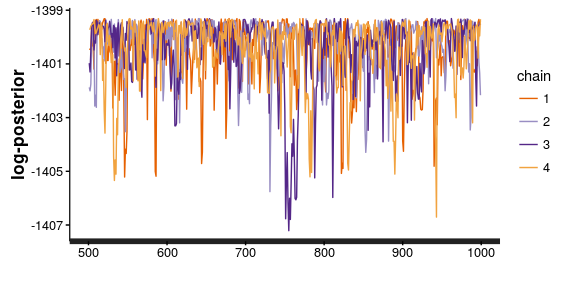Similarly, those for our parameters of interest look good: rstan::traceplot(recover_simulated2, c('alpha','mu'), ncol = 1)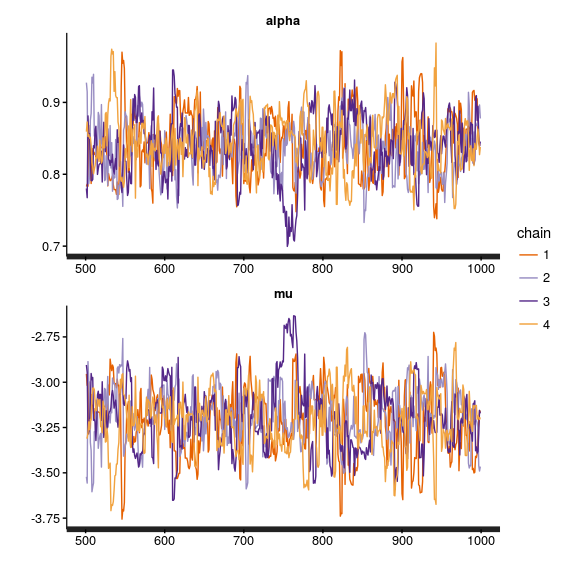##### Launch shiny-stan We could also launch shinystan to check for divergent transitions or excessive autocorrelation in the chains. This is particularly helpful when diagnosing convergence issues. if (interactive()) shinystan::launch_shinystan(recover_simulated2) ### Reviewing posterior distributions of parameters Next, we can check to see whether the model was able to recover our parameters. #### extracting parameters from the Stanfit object We use the rstan::extract() function to extract parameters from the stanfit object. E.g. to extract alpha & mu: pp_alpha <- rstan::extract(recover_simulated2,'alpha')$alpha
pp_mu <- rstan::extract(recover_simulated2,'mu')\$mu

Each of these is a 1xD vector of values, where D = the number of posterior (post-warmup) draws.

In this case, we have 2000: 4 chains * 1000 iterations / 2

For example, we can plot the posterior distribution of alpha, in context of test_alpha:

ggplot(data.frame(alpha = pp_alpha, mu = pp_mu)) +
geom_density(aes(x = alpha)) +
geom_vline(aes(xintercept = test_alpha), colour = 'red') +
ggtitle('Posterior distribution of alpha\nshowing true value in red')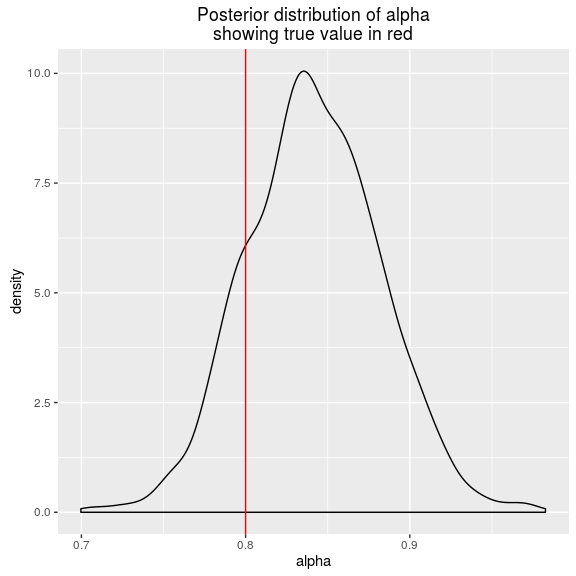and, repeat the same for mu:

ggplot(data.frame(alpha = pp_alpha, mu = pp_mu)) +
geom_density(aes(x = mu)) +
geom_vline(aes(xintercept = test_mu), colour = 'red') +
ggtitle('Posterior distribution of mu\nshowing true value in red')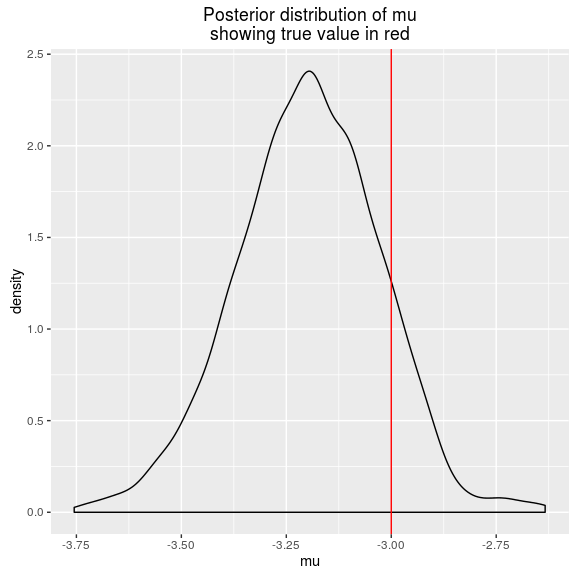The posterior estimates of the parameters do contain those used to simulate our data, but they are not necessarily at the mode of each distribution.

Also, we have a high degree of correlation between mu and alpha:

ggplot(data.frame(alpha = pp_alpha, mu = pp_mu)) +
geom_density2d(aes(x = alpha, y = mu)) +
geom_point(aes(x = test_alpha, y = test_mu), colour = 'red', size = 2) +
ggtitle('Posterior distributions of mu and alpha\nshowing true parameter values in red')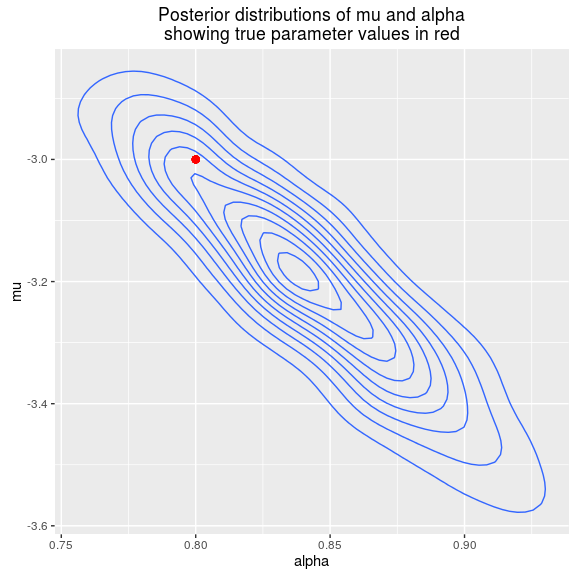It does seem like these values are on the edge of the posterior density for the parameter values.

How likely are the parameter values used to simulate our data, according to this model?

One nice thing about sampling from the full posterior is that we can compute posterior probabilities directly, by summarizing over the values.

To compute the probability of seeing a value of alpha >= 0.8:

mean(pp_alpha >= test_alpha)
##  0.841

And for mu (testing mu >= -3:

mean(pp_mu >= test_mu)
##  0.12

The joint probability of seeing this combination of parameter values, however, isn’t as encouraging:

mean(pp_mu >= test_mu & pp_alpha >= test_alpha)
##  0.018

We would need more iterations in order to estimate this with greater precision.

### Posterior predictive checks

Next we might ask whether this error in recovering the parameters used to simulate data are substantive. Perhaps we can be a little off in estimating the baseline hazard parameters, so long as our inferences about biomarkers are sustained?

To do this, we will simulate data from our posterior draws of parameters. These are called the posterior predicted values. Their distribution is the posterior predictive distribution.

(It may seem crazy to do this for simulated data, but it’s not really extra work since we will likely want to re-use this process on our observed dataset. We will take care throughout to write each step as a function to be re-used later.)

#### simulating data for each posterior draw

We can use hadley’s purrr::map2 to simulate data for each pair of mu*alpha values.

pp_newdata <-
purrr::map2(.x = pp_alpha,
.y = pp_mu,
.f = ~ weibull_sim_data(alpha = .x,
mu = .y,
n = test_n
)
)

If you’re not familiar with purrr, I recommend you inspect the resulting object.

We now have a list of D datasets, each containing a simulation according to that draw’s parameter values for mu & alpha.

Let’s plot the time to event in the posterior draws, and compare this to the test dataset we used to fit our model.

ggplot(pp_newdata %>%
dplyr::bind_rows() %>%
dplyr::mutate(type = 'posterior predicted values') %>%
bind_rows(simulated_data %>% dplyr::mutate(type = 'actual data'))
, aes(x = os_months, group = os_status, colour = os_status, fill = os_status)) +
geom_density(alpha = 0.5) +
facet_wrap(~type, ncol = 1)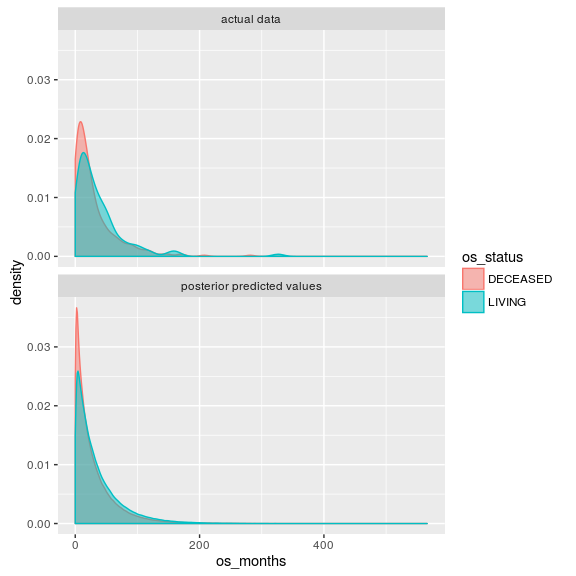These look pretty similar.

#### summarizing posterior predictive draws

Next we might ask about the posterior estimates of the survival curve. How would we estimate this?

One way (there may be several) is to:

1. compute the cumulative survival at each observed timepoint for each draw from the posterior
2. aggregate the cumulative survival estimates to discrete units of time
3. summarize the cumulative survival for each interval, over the posterior draws.

This is the method we will use here.

## cumulative survival rate for each posterior draw
pp_survdata <-
pp_newdata %>%
purrr::map(~ dplyr::mutate(., os_deceased = os_status == 'DECEASED')) %>%
purrr::map(~ survival::survfit(Surv(os_months, os_deceased) ~ 1, data = .)) %>%
purrr::map(fortify)

## summarize cum survival for each unit time (month), summarized at 95% confidence interval
pp_survdata_agg <-
pp_survdata %>%
purrr::map(~ dplyr::mutate(., time_group = floor(time))) %>%
dplyr::bind_rows() %>%
dplyr::group_by(time_group) %>%
dplyr::summarize(surv_mean = mean(surv)
, surv_p50 = median(surv)
, surv_lower = quantile(surv, probs = 0.025)
, surv_upper = quantile(surv, probs = 0.975)
) %>%
dplyr::ungroup()

Finally, we overlay the posterior predictive simulations of the survival curve with that from our original test dataset.

## km-curve for test data
test_data_kmcurve <-
fortify(
survival::survfit(
Surv(os_months, os_deceased) ~ 1,
data = simulated_data %>%
dplyr::mutate(os_deceased = os_status == 'DECEASED')
)) %>%
dplyr::mutate(lower = surv, upper = surv)

ggplot(pp_survdata_agg %>%
dplyr::mutate(type = 'posterior predicted values') %>%
dplyr::rename(surv = surv_p50, lower = surv_lower, upper = surv_upper, time = time_group) %>%
bind_rows(test_data_kmcurve %>% dplyr::mutate(type = 'actual data')),
aes(x = time, group = type, linetype = type)) +
geom_line(aes(y = surv, colour = type)) +
geom_ribbon(aes(ymin = lower, ymax = upper), alpha = 0.2) +
xlim(c(0, 200))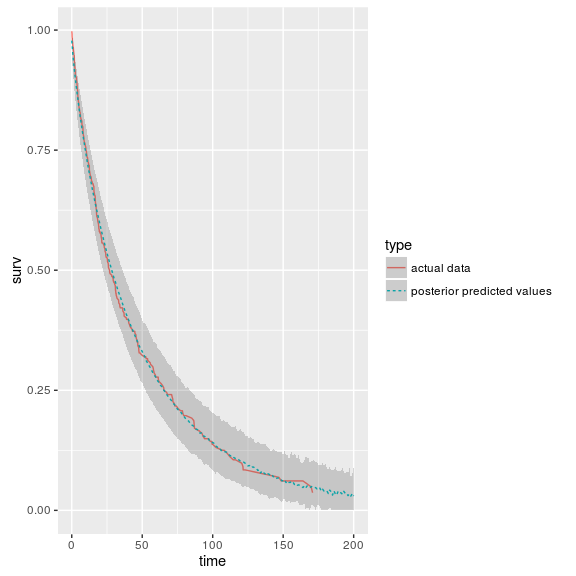Here we see that the survival curve from our original (simulated) data matches the posterior predictive density pretty closely. This is a good thing since we are working with simulated data!

#### Saving as a function

As before, we will want to wrap this in a function so that it can be reused in future steps, e.g. when we work with our TCGA data.

pp_predict_surv <- function(pp_alpha, pp_mu, n,
level = 0.9,
plot = F, data = NULL,
sim_data_fun = weibull_sim_data
) {
pp_newdata <-
purrr::map2(.x = pp_alpha,
.y = pp_mu,
.f = ~ sim_data_fun(alpha = .x, mu = .y, n = n)
)

pp_survdata <-
pp_newdata %>%
purrr::map(~ dplyr::mutate(., os_deceased = os_status == 'DECEASED')) %>%
purrr::map(~ survival::survfit(Surv(os_months, os_deceased) ~ 1, data = .)) %>%
purrr::map(fortify)

## compute quantiles given level
lower_p <- 0 + ((1 - level)/2)
upper_p <- 1 - ((1 - level)/2)

pp_survdata_agg <-
pp_survdata %>%
purrr::map(~ dplyr::mutate(.,
time_group = floor(time))) %>%
dplyr::bind_rows() %>%
dplyr::group_by(time_group) %>%
dplyr::summarize(surv_mean = mean(surv)
, surv_p50 = median(surv)
, surv_lower = quantile(surv,
probs = lower_p)
, surv_upper = quantile(surv,
probs = upper_p)
) %>%
dplyr::ungroup()

if (plot == FALSE) {
return(pp_survdata_agg)
}

ggplot_data <- pp_survdata_agg %>%
dplyr::mutate(type = 'posterior predicted values') %>%
dplyr::rename(surv = surv_p50,
lower = surv_lower,
upper = surv_upper, time = time_group)

if (!is.null(data))
ggplot_data <-
ggplot_data %>%
bind_rows(
fortify(
survival::survfit(
Surv(os_months, os_deceased) ~ 1,
data = data %>%
dplyr::mutate(
os_deceased = os_status == 'DECEASED')
)) %>%
dplyr::mutate(lower = surv,
upper = surv, type = 'actual data')
)

pl <- ggplot(ggplot_data,
aes(x = time, group = type, linetype = type)) +
geom_line(aes(y = surv, colour = type)) +
geom_ribbon(aes(ymin = lower, ymax = upper), alpha = 0.2)

pl
}

## Fitting our model to TCGA data

We are now ready to fit our model to the TCGA data. Here, we can reuse the several functions we defined in earlier steps.

wei_fit <- rstan::stan(file = stan_file,
data = gen_stan_data(clin_data),
iter = 1000,
chains = 4,
init = gen_inits
)
print(wei_fit)
## Inference for Stan model: weibull_survival_null_model.
## 4 chains, each with iter=1000; warmup=500; thin=1;
## post-warmup draws per chain=500, total post-warmup draws=2000.
##
##              mean se_mean   sd    2.5%     25%     50%     75%   97.5%
## alpha_raw   -0.01    0.00 0.01   -0.02   -0.01   -0.01   -0.01    0.00
## mu          -3.78    0.01 0.20   -4.16   -3.91   -3.78   -3.64   -3.41
## alpha        0.91    0.00 0.05    0.81    0.87    0.90    0.94    1.00
## lp__      -915.62    0.03 0.92 -918.02 -916.00 -915.32 -914.95 -914.71
##           n_eff Rhat
## alpha_raw   364 1.01
## mu          350 1.01
## alpha       365 1.01
## lp__        700 1.00
##
## Samples were drawn using NUTS(diag_e) at Wed Jun 22 22:21:19 2016.
## For each parameter, n_eff is a crude measure of effective sample size,
## and Rhat is the potential scale reduction factor on split chains (at
## convergence, Rhat=1).

## Checking convergence

In the previous section, we reviewed various ways to check convergence.

### Inspect traceplots

We then also look at the traceplot of the log-posterior. I usually check this first since it gives me a sense of the overall model fit.

rstan::traceplot(wei_fit, c('lp__'), ncol = 1)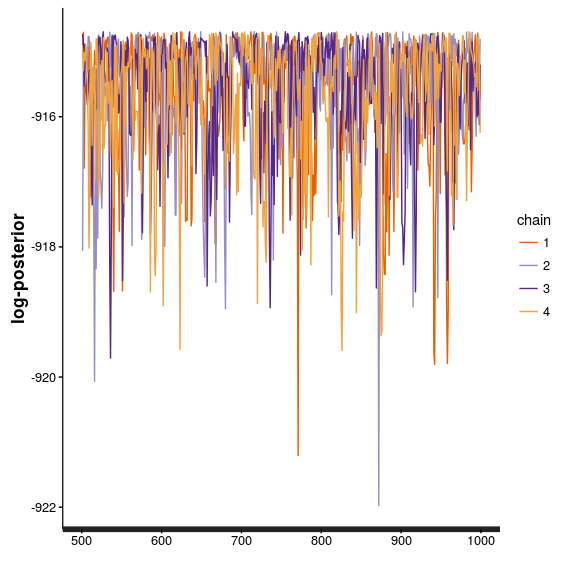And, for key parameters (in this case, alpha & mu)

rstan::traceplot(wei_fit, c('alpha','mu'), ncol = 1)